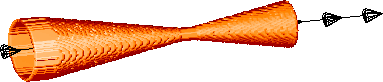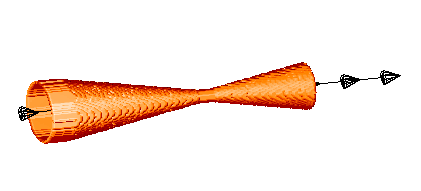## Optimization of a Two-Phase Nozzle with an ES

The animation below depicts a (1 + 1)-ES optimizing the shape of a two-phase nozzle. This is one of the 'classics' in the field. See below for a more detailed explanation.

Hans-Paul Schwefel performed the original experiments and collected the data, Uli Hermes created the 3D-model.

The nozzle to start withand the resulting nozzleIDL produced the original animation.

The resulting GIF's were put together with a tool called gifmerge by Mark Podlipec. Click here for the MPG movie.

### Additive binomial length variation of the genotype

Given the task of determining the internal shape of a two-phase jet nozzle with maximum thrust under constant starting conditions, H.-P. Schwefel was one of the first to use gene duplication and deletion.

Since no simulation model was available at the time an experimental optimization had to be performed, using a so-called (1+1) Evolution Strategy. The nozzles were built of conical pieces such that no discontinuity within the internal shape was possible. In this way every nozzle shape could be represented by its overall length and the inside diameters at the borders between the segments (every 10mm). For technical reasons the incoming diameter of the first segment had to be 32mm and the smallest diameter was fixed to 6mm resulting in an convergent-divergent structure of the nozzles.

The genotype of each nozzle had the following form:

Z1, Z2, D{-nc}, ..., D{-1}, D{1}, ..., D{nd}, where Z1 and Z2 denote the number of segments in the convergent (divergent, respectively) part of the nozzle, i.e. the number of segments in front of or behind the smallest diameter.
D{-nc}, ..., D{-1} designate the diameters in the convergent part and D{1}, ..., D{nd} those in the divergent part of the nozzle.
Since there was only a fixed number of possible diameters during each run, the problem essentially was a variable-dimensional integer optimization task. Thus, the standard mutation operator of ESs could not be used. Instead, mutation was carried out in the following form:
Z1 and Z2 were mutated with the help of a probability table comparable to an additive binomial mutation of the form: Zi' = Zi + chi, where chi + 2 ~ B(4, 0.5), i = 1, 2 The table puts a stronger emphasis on no mutation at all.
According to the new lengths Z1' or Z2', segments were added or deleted at positions chosen at random. In the case of gene duplication the additional element(s) had the same diameter as the element to be duplicated.
Finally, all diameters Di, i = -nc, ..., -1, 1, ..., nd are varied using the same probability table, this time resulting in: Di' = Di + 2 chi, i.e. the diameters change in 2mm steps.
Despite the use of a very simple (1+1) ES without recombination this first ES using gene duplication and deletion produced astonishingly good results resulting in a 'strange' nozzle shape.